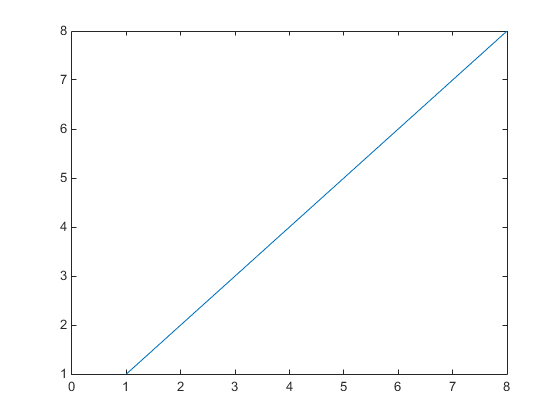## Default Values for Automatically Calculated Properties

### What Are Automatically Calculated Properties

When you create a graph, MATLAB® sets certain property values appropriately for the particular graph. These properties, such as those controlling axis limits, have an associated mode property.

The mode property determines if MATLAB calculates a value for the property (mode is `auto`) or if the property uses a specified value (mode is `manual`).

### Default Values for Automatically Calculated Properties

Defining a default value for an automatically calculated property requires two steps:

• Define the property default value

• Define the default value of the mode property as `manual`

#### Setting X-Axis Limits

Suppose you want to define default values for the x-axis limits. Because the axes `XLim` property is usually automatically calculated, you must set the associated mode property (`XLimMode`) to `manual`.

```set(groot,'defaultAxesXLim',[0 8]) set(groot,'defaultAxesXLimMode','manual') plot(1:20)```

The axes uses the default x-axis limits of `[0 8]`: